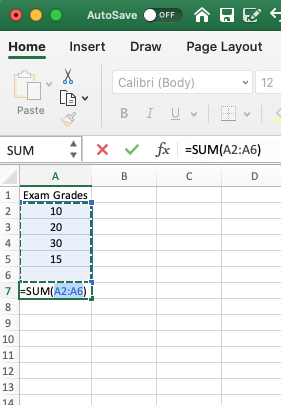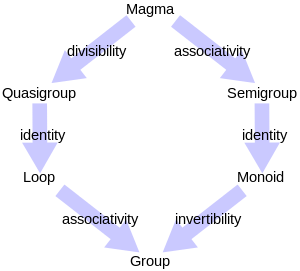1604128348

# What Is Functional Programming?

``````"On two occasions I have been asked, 'Pray, Mr. Babbage, if you put into the machine wrong figures, will the right answers come out?'...I am not able rightly to apprehend the kind of confusion of ideas that could provoke such a question."

- Charles Babbage (1864): Inventor of the Analytical Engine (the originator of digital computing)
``````

**Functional Programming **defines another programming paradigm of the ‘declarative’ (as opposed to imperative) type of programming approaches defined within Computer Science.

This article explores functional programming for beginners, using the highly popular JavaScript ES6 language for reference.

In short, functional approaches to programming are defined as applying and composing of functions, in contrast to the imperative ‘line by line’ approach to programming used by historical Computer Scientists, such as Babbage.

This begs us to ask, what are functions?

Dictionary.com unpacks a function, in Mathematical definition as…

``````Also called correspondence, map, mapping, transformation. a relation between two sets in which one element of the second set is assigned to each element of the first set, as the expression y = x^2; operator.

https://www.dictionary.com/browse/function
``````

If you haven’t studied advanced Mathematics, perhaps, beyond required schooling, or Computer Science formally (like myself); to decompose the meaning of functions in programming; it is simple to understand that we are taking a ‘set’ of defined values or ‘objects’ of data and mapping or transforming that data to another value.

We call the overarching ‘module’ that is created by transforming, for example, ‘x to y’ — a function.

Let me explain a little further herein. I will be using JavaScript ES6 functions, however I will stick to one form of expressing functions using function declarations (rather than arrow and anonymous functions), for simplicity. If you are interested in learning how to write functions in all forms within JavaScript, I suggest you take a thorough course on the subject.

## What is a function in day to day use?

It is imagined that you have grasped some of the topics and syntax of computer programming at this stage, but perhaps are not sure about what a function is and how to use a function driven approach to get your programming needs done.

If you’ve encountered Microsoft Excel in the past, with a little training, you may have been taught how to use the auto ‘sum’ feature within this program.

This feature allows the user to highlight a group of cells containing numerical values and to simply click a button to automatically compute the ‘sum’ or total of all those numbers.Excel’s SUM Feature

In Excel, this essentially serves a purpose as a pre-written function. When we write =SUM(X) and hit return in a cell in Excel, with X being the value(s) we want to total; the mapping from X to Y is undertaken under the hood.

So perhaps, in Excel, what the function composition or expressions of SUM could be determined as, might look a little like this. Using the above screenshot of Excel, the values are denoted within brackets…

``````SUM = (10 + 20 + 30 + 15)
// Returns 75
``````

The above is what you could define as an imperative approach to programming (the opposite of a functional approach). It is the function unpacked, written laboriously, but explicitly defined. In an imperative approach, we don’t refer to functions or control flow — we simply execute instructions top to bottom.

## What if we had to undertake this process without using the SUM feature within Excel for hundreds or thousands of cells containing values?

You would have a very clearly defined program, written line by line, computing everything you explicitly defined, but perhaps it would take a much longer period of time than simply defining what the function SUM does and then using it once, or as many times as required.

This is, at it’s heart, what functional programming is all about…

To conclude the semantics of definition, a function is basically a transformation of values from X to Y. As we saw in SUM, a set of number or numbers (X) to a single total (Y). To add to this, we typically ‘return’ a value (Y) as a result of the function — so that the function serves a purpose in the program.

In programming parlance, functions are typically declared and ‘named’ — as is ‘SUM’ the name of a computation to total a set of values within Excel — in various computer programming languages, you can define a function to do whatever computation (or transformation) you wish and give it an apt name.

We aren’t limited to just summing numbers — we can write functions to merge arrays, unpack objects, iterate and filter values into new data structures and so on and so forth, the limit is really what the language is capable of doing with the given data structures.

#es6 #javascript #functional-programming #developer

## Buddha Community1595593200

## Functional Programming

Functional Programming is a Declarative style of Programming Paradigm for writing computer programs.
But, What are Functions ?
Functions in general, applies computation on given input and returns the output. It relates input to an output.
f(x) = x + 2;
f(1) = 1 + 2 = 3;
f(2) = 2 + 2 = 4;
Above mentioned is a simple function that adds 2 to the input value and returns output. It relates value [1,2] => [3,4]. Similarly, a function in computer programming is a block of instruction that performs computation on given input and returns the output.
Functional Programming is such a style of writing computer programs using these tiny functions that works together and perform required computation.
Functions in Functional Programming
The philosophy of functional programming is to maintain certain characteristics while writing functions in a computer program. These characteristics are the fundamental nature of functional programming that describe what shall be the behaviour of a function. These are as follows :
Declarative
A function must be declarative, it simply tells what to compute, without specifying how to compute it.
f(x) = x * 4; 👍
Declarative function that tells to multiply input by 4;
f(x) = { y = x + x; z = x + x; return y + z;} 👎
Non-Declarative function that specify how to multiply input by 4;
Pure
A function must give the same output for a given input value, at any period of time. It is not dependent upon anything outside the function definition.
f(x) = It’s never too late; 👍
Pure function that will always return It’s never too late
f(x) = If today’s Friday or Saturday then It’s never too late else It’s late. 👎
Impure function that consider day for returning value. The value is not predictable. It can change. So a function that performs anything which is unpredictable is not a pure function. The condition or the execution is dynamic or unfixed in an impure function.

#development #functional-programming #software #software-programming #declarative-programming #function1598093520

## Are functions from programming really functions?

If you are reading this, then most probably you already know quite well what functions are in programming. A function is quite a common and spread programming construct that is present in almost all programming languages.

Generally, a function is a block of code that takes some parameters from outside, executes some operations in which these parameters may be used, then it returns an output value. Actually, in many programming languages functions are allowed to not return something or to return multiple values, not only one. But these cases can be also be represented, for the sake of generality, as only one value. For the “no return” case we can use a special value to represent that (in Python that special value is None; whenever you don’t return something from a function it’s returned None). And for the case of more values, we can use a vector of multiple values as a single object.

For example, in Python a function definition looks like this:

``````def func_name(param1, param2, ...):

## do some stuff with input parameters
return output_value
``````

Now, the question I want to ask: Are these functions from programming true mathematical functions?

Well…, let’s first recall what a mathematical function is.

In mathematics, a function is just a mapping from a set A to a set B, in which any element from A has only one associated element in B.

#python #programming #function #mathematics #functional-programming1596300660

## Functional Programming Series (2): What Is a Monoid?

For those interested in functional programming, I’ll talk about monoids and why they’re very important to understand ahead of time.

Don’t get confused: This isn’t monad — it’s monoid. I’m pretty sure you already know of monoids and you use them almost every day — you just didn’t know the term for them.

# Identity Function

Let’s assume there’s a function named `identity` that takes `A` and returns `A`.

``````const identity: <A>(a: A): A => a;

interface Student {
name: string;
age: number;
}
identity<number>(3) // 3
identity<string>('hello') // hello
identity<Student>({
name: 'Bincent',
age: 5
}); // { name: 'Bincent', age: 5 }
``````

In functional programming, this useless function (seems useless) is an important factor for many other concepts (such as monoids) that we’re about to talk about.Basically, a monoid is a set of elements that holds the rules of the semigroup and the identity-element rule.

If `S` is a set of elements, `a` is a member of `S`, and `·` is a proper binary operation, `a·e = e·a ∈ S` must be satisfied to be a monoid.

``````Identity: a ∈ S, a·e = e·a = a ∈ S
``````

Some documentation calls this using the number 1 and the any alphabet in subscript — for example, 1x referring to the identity on the variable x. Or some documentation uses just a single alphabet letter, such as or e.

That’s all there is to know about monoids, let’s practice with some simple examples.

#typescript #programming #functional-programming #javascript #coding #function1600455600

## What is functional programming?

In simple terms, functional programming is a software development style that places a major emphasis on the use of functions. You might say, “Well, I already use functions daily, what’s the difference?” Well, it’s not a matter of just applying functions to come up with a result. The goal, rather, is to abstract control flows and operations on data with functions in order to avoid side effects and reduce mutation of state in your application.

#programming #javascript #functional #web-development #functional-programming1605017502

## The Difference Between Regular Functions and Arrow Functions in JavaScript

Other then the syntactical differences. The main difference is the way the this keyword behaves? In an arrow function, the this keyword remains the same throughout the life-cycle of the function and is always bound to the value of this in the closest non-arrow parent function. Arrow functions can never be constructor functions so they can never be invoked with the new keyword. And they can never have duplicate named parameters like a regular function not using strict mode.

###### Here are a few code examples to show you some of the differences
```this.name = "Bob";
const person = {
name: “Jon”,
```<span style="color: #008000">// Regular function</span>
func1: <span style="color: #0000ff">function</span> () {
console.log(<span style="color: #0000ff">this</span>);
},

<span style="color: #008000">// Arrow function</span>
func2: () =&gt; {
console.log(<span style="color: #0000ff">this</span>);
}
```
}
person.func1(); // Call the Regular function
// Output: {name:“Jon”, func1:[Function: func1], func2:[Function: func2]}
person.func2(); // Call the Arrow function
// Output: {name:“Bob”}```

###### The new keyword with an arrow function
```const person = (name) => console.log("Your name is " + name);
const bob = new person("Bob");
// Uncaught TypeError: person is not a constructor```

###### If you want to see a visual presentation on the differences, then you can see the video below:

#arrow functions #javascript #regular functions #arrow functions vs normal functions #difference between functions and arrow functions